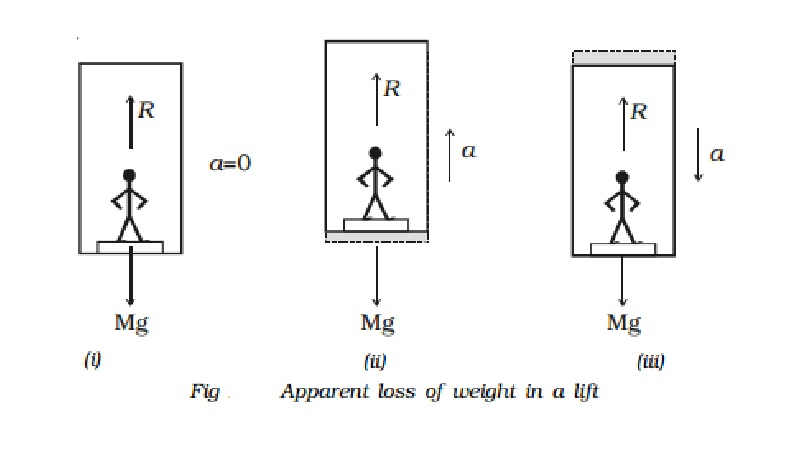Home | | Physics | Applications of Newton s third law of motion

# Applications of Newton s third law of motionLet us consider a man of mass M standing on a weighing machine placed inside a lift. The actual weight of the man = Mg. This weight (action) is measured by the weighing machine and in turn, the machine offers a reaction R. This reaction offered by the surface of contact on the man is the apparent weight of the man.

Applications of Newton's third law of motion

(i) Apparent loss of weight in a lift

Let us consider a man of mass M standing on a weighing machine placed inside a lift. The actual weight of the man = Mg. This weight (action) is measured by the weighing machine and in turn, the machine offers a reaction R. This reaction offered by the surface of contact on the man is the apparent weight of the man.

Case (i)

When the lift is at rest:

The acceleration of the man = 0

Therefore, net force acting on the man = 0

From Fig. (i),        R - Mg = 0 (or)    R = MgThat is, the apparent weight of the man is equal to the actual weight.

Case (ii)

When the lift is moving uniformly in the upward or downward direction:

For uniform motion, the acceleration of the man is zero. Hence, in this case also the apparent weight of the man is equal to the actual weight.

Case (iii)

When the lift is accelerating upwards:

If a be the upward acceleration of the man in the lift, then the net upward force on the man is F = Ma

From Fig (ii), the net force

= R - Mg = Ma (or) R = M ( g + a )

Therefore, apparent weight of the man is greater than actual weight.

Case (iv)

When the lift is accelerating downwards:

Let a be the downward acceleration of the man in the lift, then the net downward force on the man is F = Ma

From Fig. (iii), the net force

= Mg - R = Ma (or)    R = M (g + a)

Therefore, apparent weight of the man is less than the actual weight.

When the downward acceleration of the man is equal to the acceleration due to the gravity of earth, (i.e) a = g

R = M (g - g) = 0

Hence, the apparent weight of the man becomes zero. This is known as the weightlessness of the body.

(ii) Working of a rocket and jet plane

The propulsion of a rocket is one of the most interesting examples of Newton's third law of motion and the law of conservation of momentum.

The rocket is a system whose mass varies with time. In a rocket, the gases at high temperature and pressure, produced by the combustion of the fuel, are ejected from a nozzle. The reaction of the escaping gases provides the necessary thrust for the launching and flight of the rocket.

From the law of conservation of linear momentum, the momentum of the escaping gases must be equal to the momentum gained by the rocket. Consequently, the rocket is propelled in the forward direction opposite to the direction of the jet of escaping gases. Due to the thrust imparted to the rocket, its velocity and acceleration will keep on increasing. The mass of the rocket and the fuel system keeps on decreasing due to the escaping mass of gases.

Study Material, Lecturing Notes, Assignment, Reference, Wiki description explanation, brief detail
11th 12th std standard Class Physics sciense Higher secondary school College Notes : Applications of Newton s third law of motion |